# Optimized pulse width modulation pattern strategy for three-dimensional profilometry

156

Optimized pulse width modulation pattern strategy for three-dimensional profilometry with projector defocusing

Chao Zuo, Qian Chen, Shijie Feng, Fangxiaoyu Feng, Guohua Gu, and Xiubao Sui

Jiangsu Key Laboratory of Spectral Imaging & Intelligent Sense,

Nanjing University of Science and Technology, Nanjing, Jiangsu Province 210094, China

Abstract

Three-dimensional profilometry by sinusoidal fringe projection using phase-shifting algorithms is usually distorted by the nonlinear intensity response of commercial video projectors. To overcome this problem, several methods including sinusoidal pulse width modulation (SPWM) were proposed to generate sinusoidal fringe patterns with binary ones by defocusing the project to some certain extent. However, the residual errors are usually non-negligible for highly accurate measurement fields, especially when the defocusing level is insufficient. In this work, we propose two novel methods to further improve the defocusing technique. We find that by properly optimizing SPWM patterns according to some criteria, and combining SPWM technique with four-step phase-shifting algorithm, the dominant undesired harmonics will have no impact on the phase obtained. We also propose a new sinusoidal fringe generation technique called tripolar SPWM, which can generate ideal sinusoidal fringe patterns with a very small degree of defocusing. Simulations and experiments are presented to verify the performance of these two proposed techniques.

Files

PDF

Citations

Chao Zuo, Qian Chen, Shijie Feng, Fangxiaoyu Feng, Guohua Gu, and Xiubao Sui, "Optimized pulse width modulation pattern strategy for three-dimensional profilometry with projector defocusing," Appl. Opt. 51, 4477-4490 (2012).

Results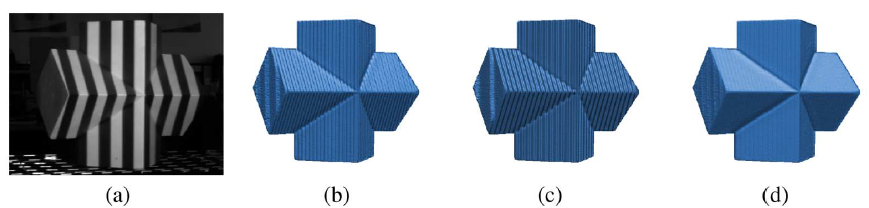Fig. 1. 3D measurement results of a plaster geometric model using different patterns (fringe pitch 60 pixels) with different phase-shifting algorithms when the projector is slightly defocused. The first row shows the tested object with SBM pattern (a), the 3D results of three-, four-, and five-step phase-shifting algorithms using SBM patterns (b)–(d).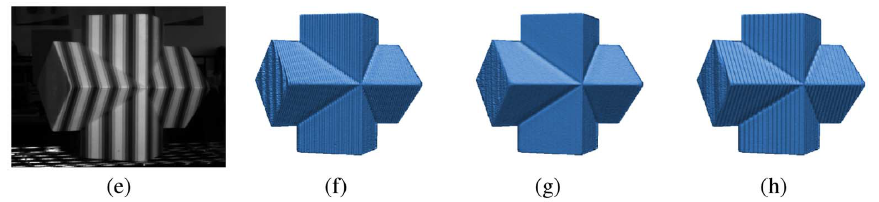The second row shows the tested object with SPWM pattern (f c= 8f 0) (e), the 3-D results of three-, four-, and five-steps phase-shifting algorithms using SPWM patterns (f c= 8f 0) (f–h).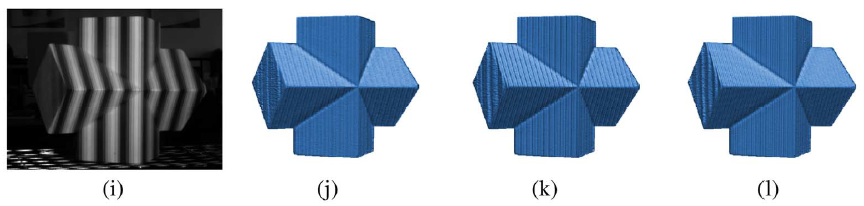The third row shows the tested object with SPWM pattern (f c = 9f 0) (i), the 3D results of three-, four-, and five-steps phase-shifting algorithms using SPWM patterns (f c = 9f 0) (j)–(l).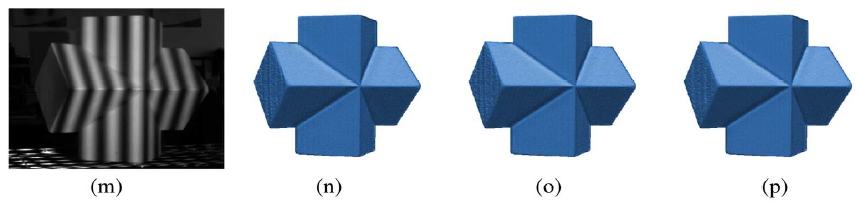The forth row shows the tested object with tripolar SPWM pattern ( f c = 8f 0) (m), and the 3D results of three-, four-, and five-step phase-shifting algorithms using tripolar patterns ( f c = 8f 0) (n-p).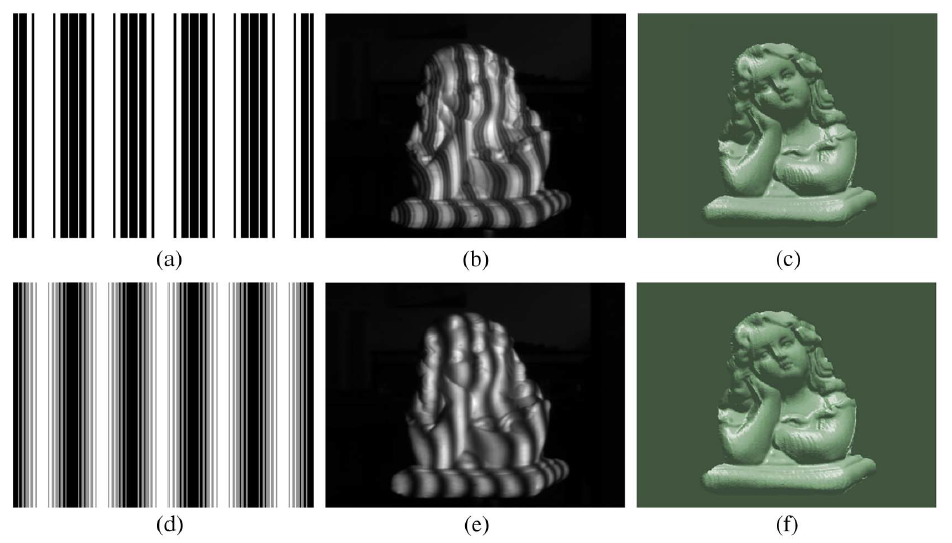Fig. 2. 3D shape measurement of a complex sculpture using the proposed techniques (fringe pitch 48 pixels). (a) One of SPWM pattern with f c = 6f 0; (b) the measured object with the slightly defocused SPWM pattern; (c) 3D result with the SPWM plus four step phase-shifting method; (d) one of tripolar SPWM pattern with f c = 7f 0; (e) the measured object with the slightly defocused tripolar SPWM pattern; (f) 3D result with the tripolar SPWM plus three-step phase-shifting method.

Methods

Table 1. Sensitivity of Different Phase-Shifting Algorithms to Harmonics.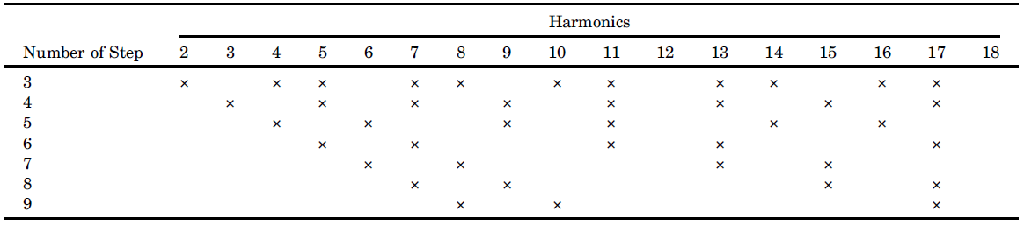To analyze how each harmonic frequency component affects the calculated phase for different phase-shifting algorithms, we just added one specific harmonic component to the ideal sinusoid wave signals one time. Then we compared the calculated phase with the correct one, and we would know whether we can get a distortion-free phase even when the particular harmonic component exists. Table 1 summarizes the results.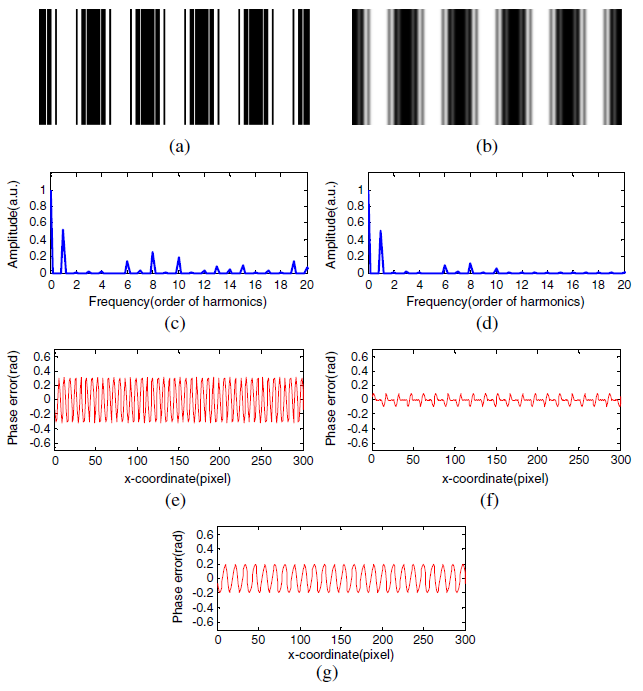Fig. 1. Simulation results of SPWM (f c = 8f 0) using three to five-step phase-shifting algorithms. (a) The original SPWM pattern; (b) the Gaussian smoothed version of (a); (c) frequency spectrum of (a); (d) frequency spectrum of (b); (e) the phase error of three-step algorithm (RMS: 0.2319 rad); (f) the phase error of four-step algorithm (RMS: 0.0436 rad); (g) the phase error of five-step algorithm(RMS: 0.1396 rad).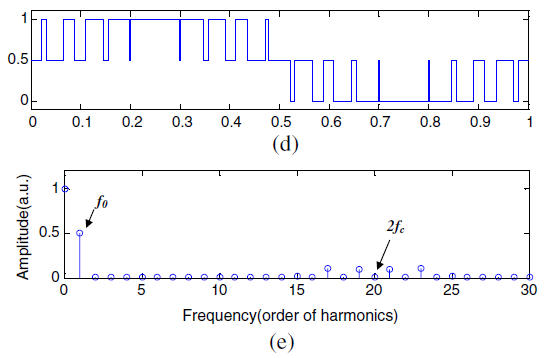Fig.2 shows how the tripolar SPWM pattern is generated (f c = 10f 0). First, two high-frequency triangular carriers displaced by π shift in phase are compared with desired a sinusoidal pattern to get two bipolar SPWM patterns. Note the second bipolar SPWM pattern corresponding to the π shifted triangular carrier (shown in red) should be inversed; i.e., when the intensity value of the sinusoidal wave form is less than the triangular waveform, the value of SPWM pattern is “1”; otherwise, the intensity value is “0.” Then the resultant tripolar SPWM waveform is the difference of the two bipolar SPWM patterns.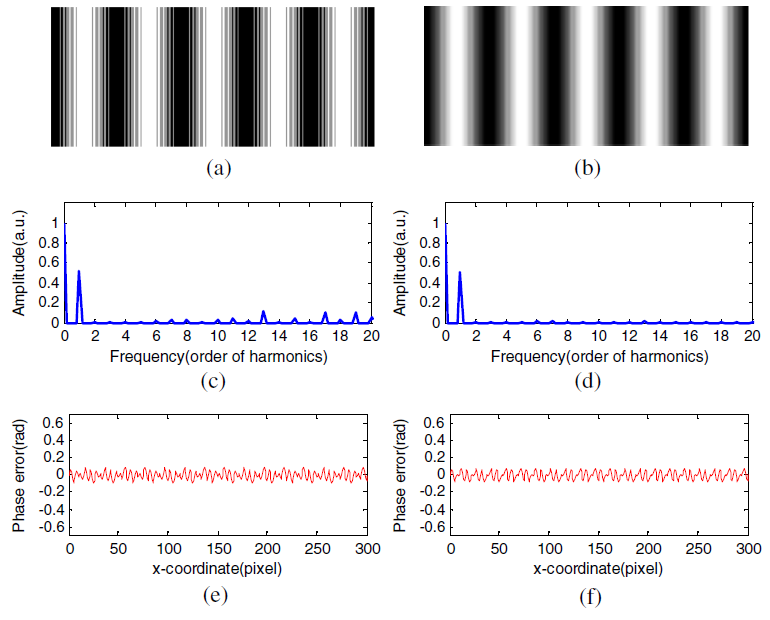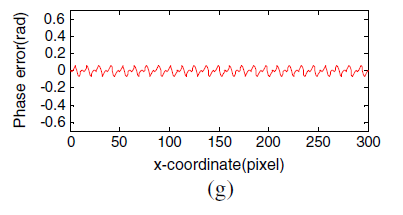Fig. 3. Simulation results of tripolar SPWM(f c = 8f 0) using three to five-step phase-shifting algorithms. (a) The originaltripolar SPWM pattern; (b) the Gaussian smoothed version of (a) (filter size 8 pixels, standard deviation 1.5 pixels); (c) frequency spectrumof (a); (d) frequency spectrum of (b); (e) the phase error of three-step algorithm (RMS: 0.0488 rad); (f) the phase error of four-step algorithm(RMS: 0.0468 rad); (g) the phase error of five-step algorithm (RMS: 0.0389 rad).

------------------------------------------------

Contact

Chao Zuo

Associate professor at the school of Electronic and Optical Engineering

Email: surpasszuo@163.com

Nanjing University of Science and Technology, Jiangsu Province (210094), P.R.China

Qian Chen

Dean of the school of Electronic and Optical Engineering

Email: chenqian@njust.edu.cn

Nanjing University of Science and Technology, Jiangsu Province (210094), P.R.China

Shijie Feng

Ph.D. Candidate of NJUST

Email:geniusshijie@163.com ( or 311040574@njust.edu.cn)

Nanjing University of Science and Technology, Jiangsu Province (210094), P.R.China